# Name (First and Last): Stoichiometry and Mass Relationships Report Pages Obtain values from the pictures found...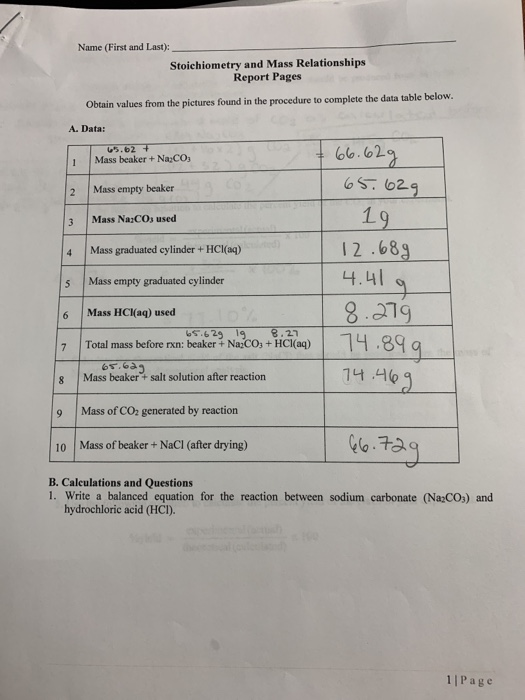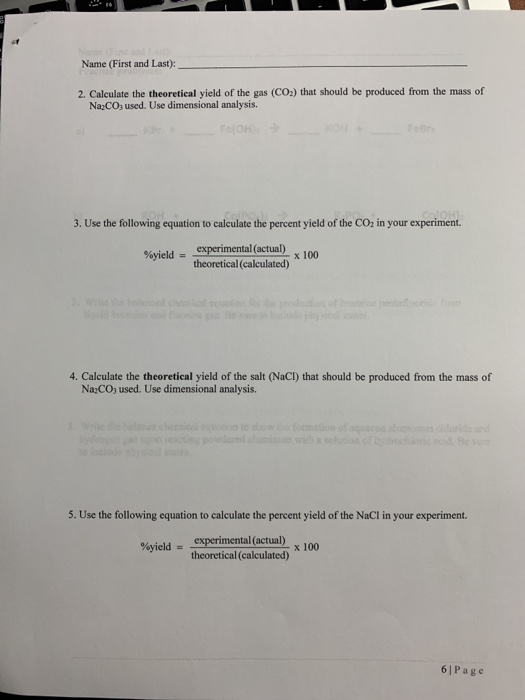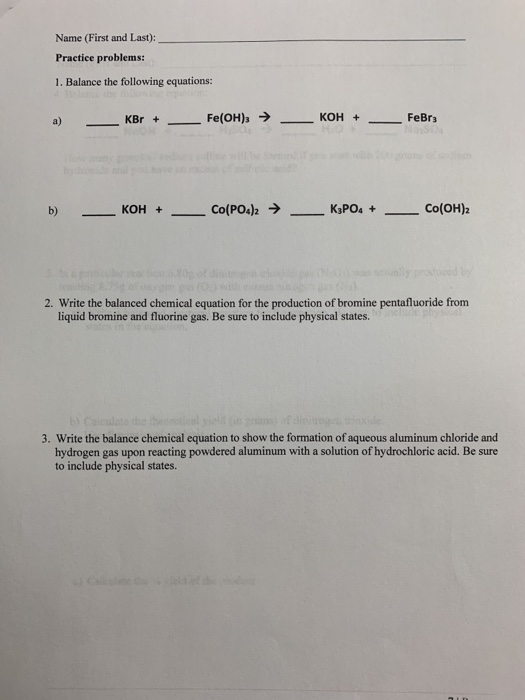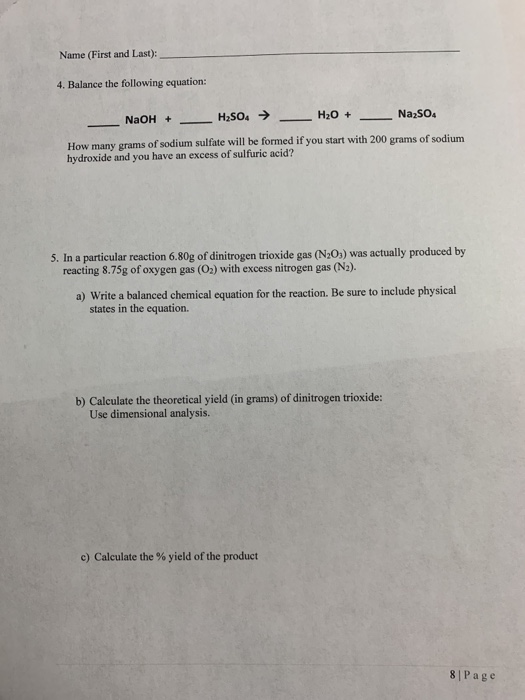Name (First and Last): Stoichiometry and Mass Relationships Report Pages Obtain values from the pictures found in the procedure to complete the data table below A. Data: 65.62 + Mass beaker + NaCO3 + 66.62g 65.62g 2 Mass empty beaker 3 Mass Na:COs used 19. 4 Mass graduated cylinder + HCl(aq) 5 Mass empty graduated cylinder 12.689 4.41 g 8. 279 1 74.899 Mass HCl(aq) used 65.629 19 8.27 Total mass before rxn: beaker + Nazco, + HCl(aq) 65.623 8 Mass beaker + salt solution after reaction 74.469 9 Mass of CO2 generated by reaction Mass of beaker + NaCl (after drying) 66.729 B. Calculations and Questions 1. Write a balanced equation for the reaction between sodium carbonate (Na2CO3) and hydrochloric acid (HCI). 1 | Page
Name (First and Last): 2. Calculate the theoretical yield of the gas (CO2) that should be produced from the mass of Na2COused. Use dimensional analysis. 3. Use the following equation to calculate the percent yield of the CO2 in your experiment %yield = experimental (actual) theoretical (calculated) d elet 4. Calculate the theoretical yield of the salt (NaCl) that should be produced from the mass of Na2CO3 used. Use dimensional analysis. 5. Use the following equation to calculate the percent yield of the NaCl in your experiment. %yield = experimental (actual) x 100 theoretical (calculated) 6 Page
Name (First and Last): Practice problems: 1. Balance the following equations: a) KBr + Fe(OH)3 → __ KOH + __ FeBry KOH + __ Co(POc)2 → __ K3PO4 + — Co(OH)2 2. Write the balanced chemical equation for the production of bromine pentafluoride from liquid bromine and fluorine gas. Be sure to include physical states. 3. Write the balance chemical equation to show the formation of aqueous aluminum chloride and hydrogen gas upon reacting powdered aluminum with a solution of hydrochloric acid. Be sure to include physical states.
Name (First and Last): 4. Balance the following equation: NaOH + - H2SO4 → - H2O + Na2SO4 How many grams of sodium sulfate will be formed if you start with 200 grams of sodium hydroxide and you have an excess of sulfuric acid? 5. In a particular reaction 6.80g of dinitrogen trioxide gas (N203) was actually produced by reacting 8.75g of oxygen gas (O2) with excess nitrogen gas (N2). a) Write a balanced chemical equation for the reaction. Be sure to include physical states in the equation. b) Calculate the theoretical yield (in grams) of dinitrogen trioxide: Use dimensional analysis. c) Calculate the % yield of the product 8 Page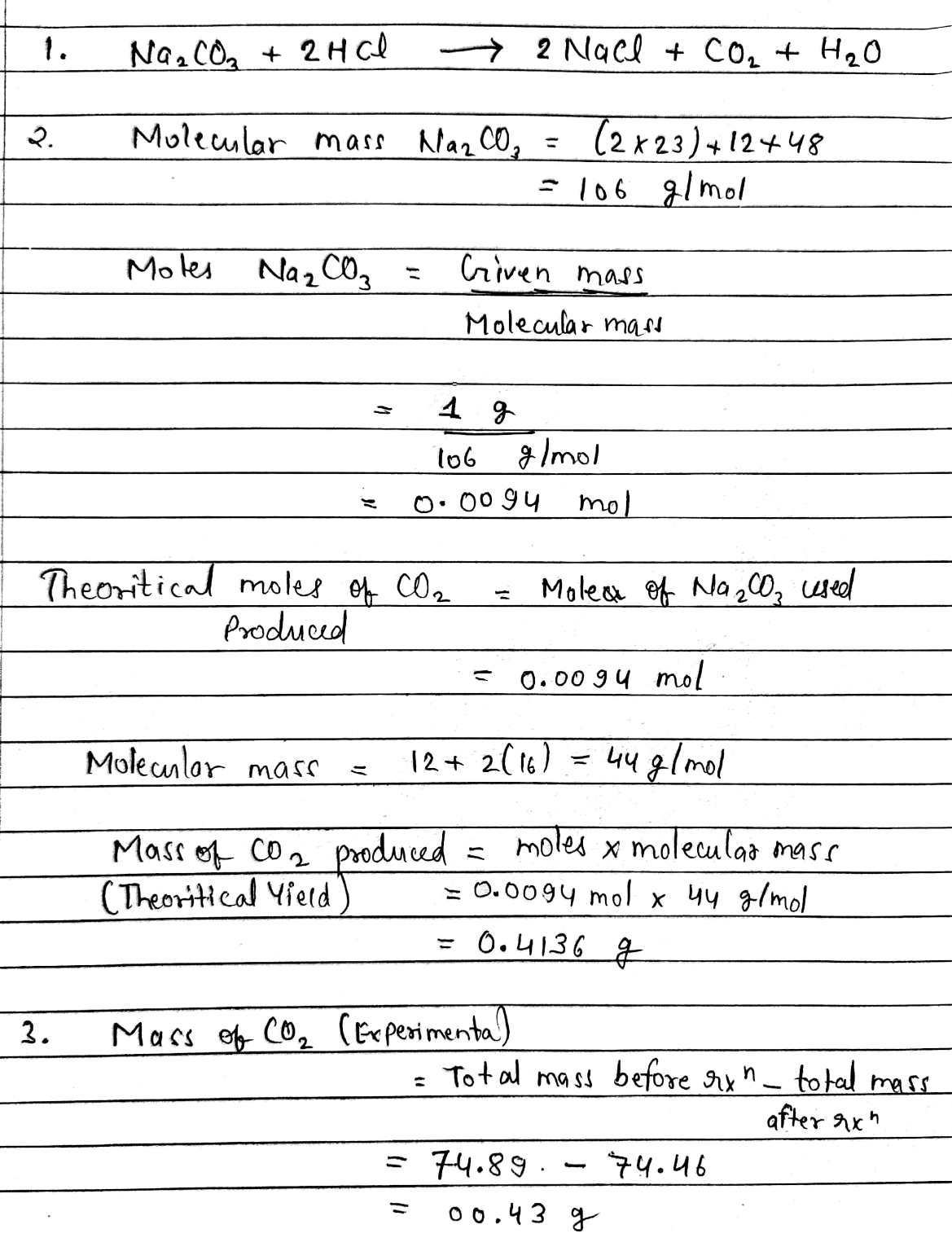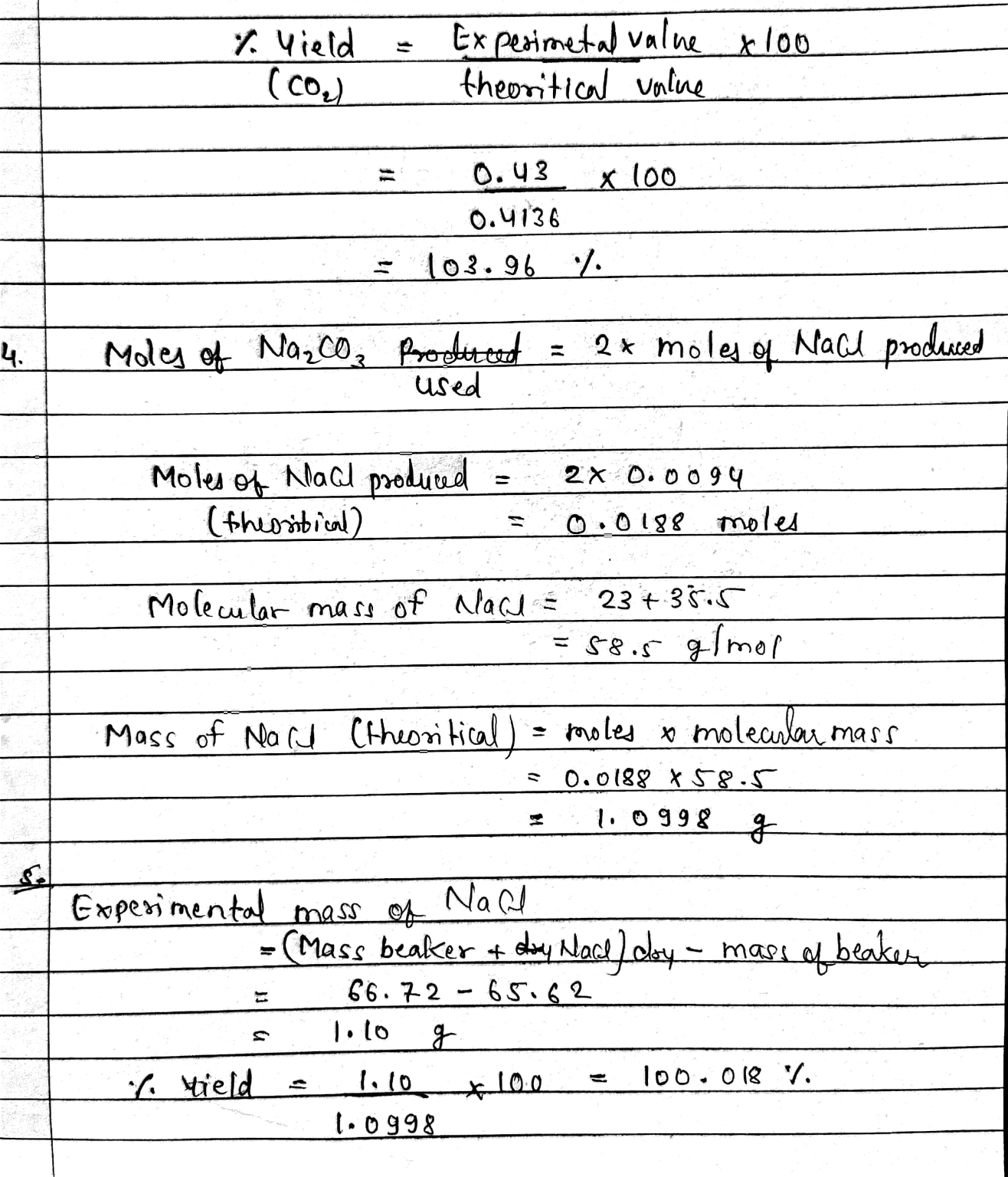Moles of sodium carbonate used in reaction =0.0094 moles

Theoretical mass of CO​​​​​2 produced = 0.4136 g

Theoretical mass of NaCl produced = 1.0998 g

Please send multiple question separate as we have time limit to answer.

#### Earn Coin

Coins can be redeemed for fabulous gifts.

Similar Homework Help Questions
• ### Name (First and Last Practice problems 1. Balance the following equations: *) - KB - Fe(OH)...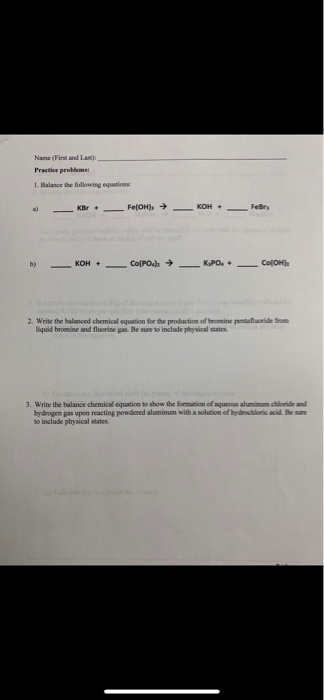Name (First and Last Practice problems 1. Balance the following equations: *) - KB - Fe(OH) → — KOH. - Fer b) - KOH + Co[POR → K.PO. COOH 2. Write the balanced chemical equation for the production of homine pentru defe liquid bromine and fluorine pas. Be sure to include physical states 3. Write the balance chemical equation to show the formation of aques aluminum chloride and hydrogen pas upon reacting powdered aluminum with a solution of hydrochloric acid....

• ### quellion) 1. Balance the following equations: - KBr + Fe(OH)3 + KOH + - FeBrz b)...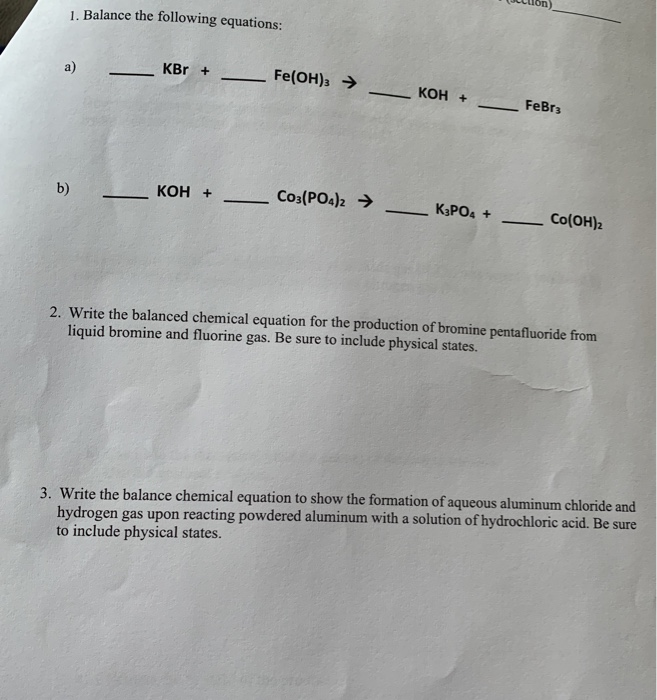quellion) 1. Balance the following equations: - KBr + Fe(OH)3 + KOH + - FeBrz b) - KOH + - Co3(PO4)2 → K3PO4 + CO(OH)2 2. Write the balanced chemical equation for the production of bromine pentafluoride from liquid bromine and fluorine gas. Be sure to include physical states. 3. Write the balance chemical equation to show the formation of aqueous aluminum chloride and hydrogen gas upon reacting powdered aluminum with a solution of hydrochloric acid. Be sure to include...

• ### Data Table 1. Mass of Beaker     49.93g 2. Mass of beaker + Na2CO3 50.95g 3....

Data Table 1. Mass of Beaker     49.93g 2. Mass of beaker + Na2CO3 50.95g 3. Mass of Na2CO3 added into the beaker (line 2-line 1) 1.02g 4. Mass of graduated cylinder with 3M HCl solution 12.68g 5. Mass of graduated cylinder without 3M HCl solution 4.44g 6. Mass of 3M HCL solution added into the beaker (line4-line5) 8.24g 7. Mass of beaker + reactants (HCl+ Na2CO3; line 2+line 6) 59.19g 8. Mass of beaker + products without CO2 (after...

• ### EXPERIMENT # 14 NAME: jerrika Foster TABLE SALT FROM SODA ASH REPORT FORM 29.87 31.08 8...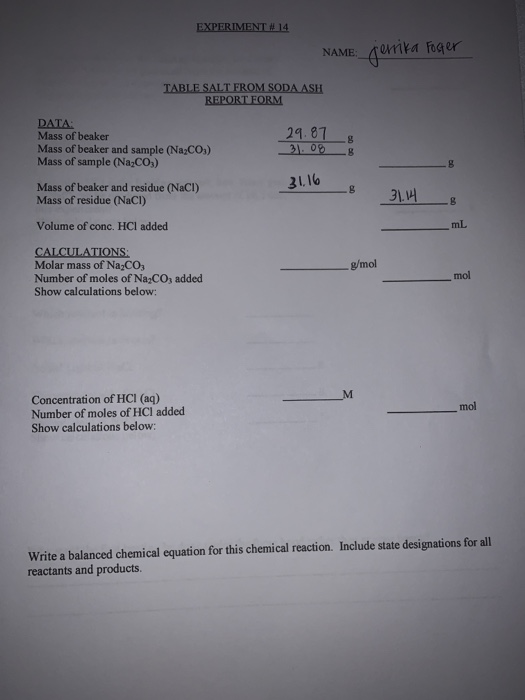EXPERIMENT # 14 NAME: jerrika Foster TABLE SALT FROM SODA ASH REPORT FORM 29.87 31.08 8 8 DATA: Mass of beaker Mass of beaker and sample (Na2CO:) Mass of sample (Na,CO;) Mass of beaker and residue (NaCl) Mass of residue (NaCl) 8 31.16 B 31.14 B Volume of conc. HCI added mL g/mol CALCULATIONS Molar mass of Na.Co Number of moles of Na2CO3 added Show calculations below: mol M mol Concentration of HCI (aq) Number of moles of HCI added...

• ### NAME garita herr TABLE SALLROMESCOASE DATA Mansehen 10 2016 31 Volume of one CALCULATIONS Melasofa.co Number...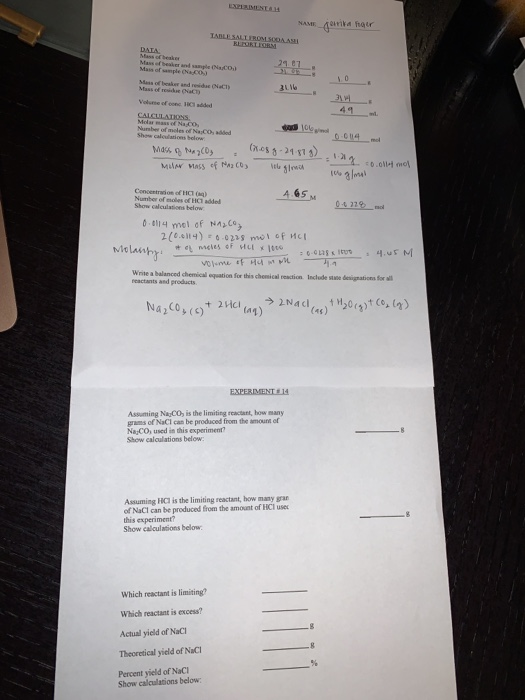NAME garita herr TABLE SALLROMESCOASE DATA Mansehen 10 2016 31 Volume of one CALCULATIONS Melasofa.co Number of miles of coded Show can be Mass of NazCO, (7.08 g - 29.87g) MUN MASS of NA legri 12. 011-mol 100 gloril M Concentration of HCM 4.65 Number of soles of Hadded Show Gallons below 0.0114 mol of Na2CO3 2 (0.0114) = 0.0228 mil of HCl Molanty. #mcles of Hulle Write a balanced chemical equation for this chemical reaction. Include e designations for...

• ### Table Salt from Baking Soda Name: Date: Partner: DATA: Mass of beaker 95.85. Mass of beaker...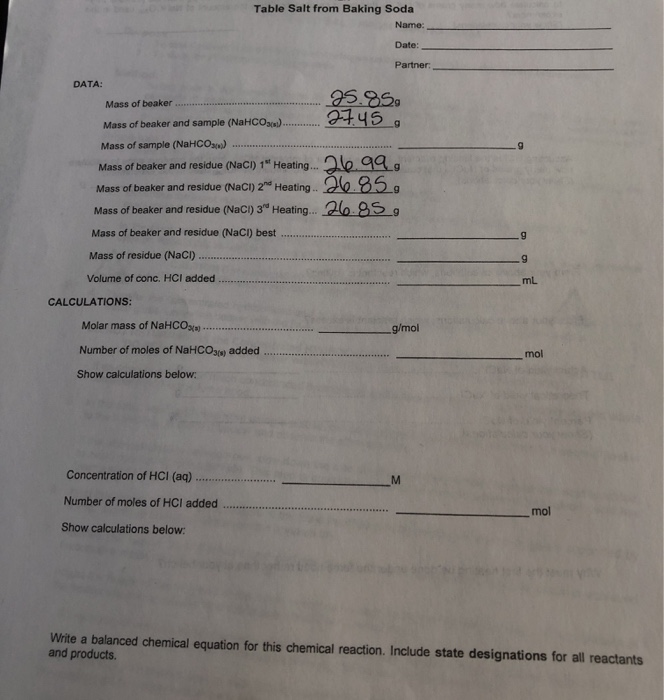Table Salt from Baking Soda Name: Date: Partner: DATA: Mass of beaker 95.85. Mass of beaker and sample (NaHCO3) 17.45. Mass of sample (NaHCO3) Mass of beaker and residue (NaCl) 1" Heating... :26 99, Mass of beaker and residue (NaCl) 2 Heating.. 26.85, Mass of beaker and residue (NaCl) 3* Heating... 26.959 Mass of beaker and residue (NaCl) best g Mass of residue (NaCl) 9 Volume of conc. HCI added ml CALCULATIONS: g/mol Molar mass of NaHCO3 Number of moles...

• ### Write a balanced equation for the reaction between sodium carbonate (Na2CO3) and hydrochloric acid (HCI). Calculate...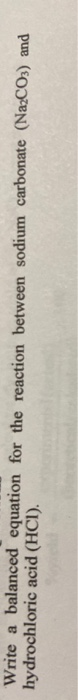Write a balanced equation for the reaction between sodium carbonate (Na2CO3) and hydrochloric acid (HCI). Calculate the theoretical yield of the gas (CO2) that should be produced from the mass of Na2CO3 used. Use dimensional analysis.

• ### Procedure EXPERIMENT #14 NAME: jerrika Foster TABLE SALT FROM SODA ASH REPORT FORM 29.87 31.08 8...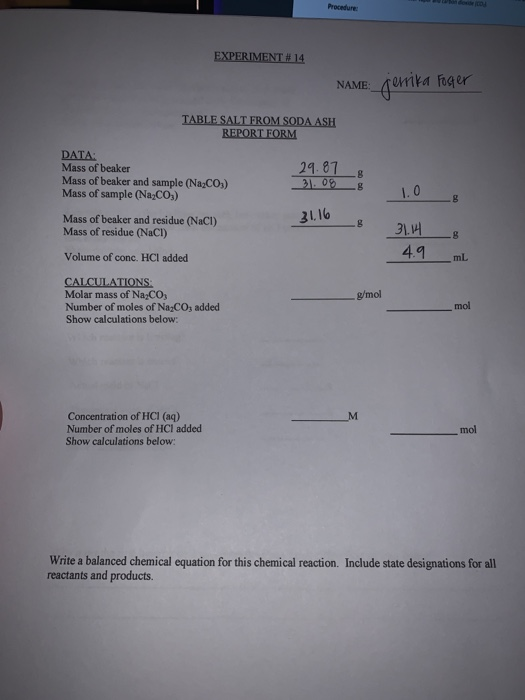Procedure EXPERIMENT #14 NAME: jerrika Foster TABLE SALT FROM SODA ASH REPORT FORM 29.87 31.08 8 8 DATA: Mass of beaker Mass of beaker and sample (Na2CO3) Mass of sample (Na2CO3) Mass of beaker and residue (NaCl) Mass of residue (NaCl) 1.0 8 31.16 B 8 31.14 4.9 Volume of conc. HCI added mL CALCULATIONS Molar mass of Na2CO3 Number of moles of Na2CO3 added Show calculations below: g/mol mol M Concentration of HCI (aq) Number of moles of HCI...

• ### may you please provide details on how you recieved the answer thank you. 3 pages. Chem...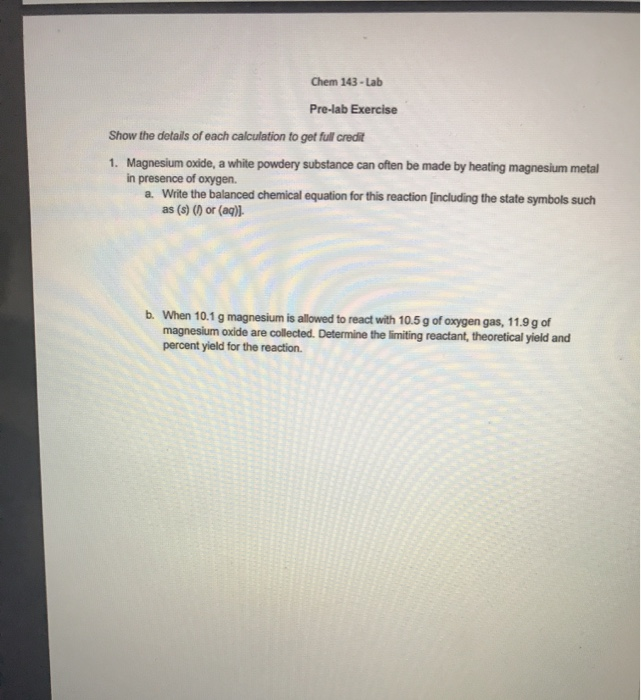may you please provide details on how you recieved the answer thank you. 3 pages. Chem 143 - Lab Pre-lab Exercise Show the details of each calculation to get full credit 1. Magnesium oxide, a white powdery substance can often be made by heating magnesium metal in presence of oxygen. a. Write the balanced chemical equation for this reaction (including the state symbols such as (s) () or (aq)] b. When 10.1 g magnesium is allowed to react with 10.5g...

• ### Exact mass of sodium bicarbonate: 2.110g Molar mass of sodium bicarbonate: 84.007 g/mol Molar mas...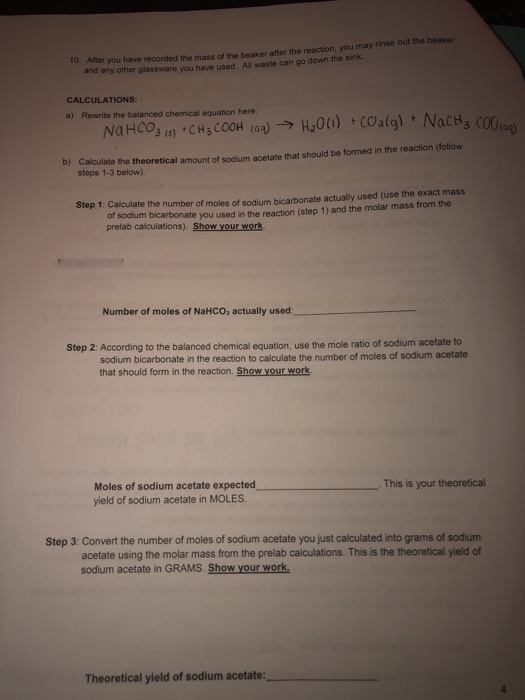Exact mass of sodium bicarbonate: 2.110g Molar mass of sodium bicarbonate: 84.007 g/mol Molar mass of sodium acetate: 82.034 g/mol After you have recorded and any other glassware you have used. All waste can go down the sink. the mass of the beaker after the reaction, you may rinse out the beaker 10. CALCULATIONS: a) Rewrite the balanced chemical equation here Calculate the theoretical amount of sodium acetate that should be formed in the reaction (follow steps 1-3 below). b)...

Free Homework App